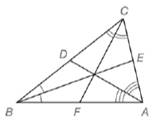Chapter 5.6, Problem 41EElementary Geometry For College St...

7th Edition
Alexander + 2 others
ISBN: 9781337614085

Solutions

Chapter
SectionElementary Geometry For College St...

7th Edition
Alexander + 2 others
ISBN: 9781337614085
Textbook Problem

In the figure, the angle bisectors of Δ A B C intersect at a point in the interior of the triangle. If B C = 5 , B A = 6 , and C A = 4 , find:a) C D and D B (HINT: Use Theorem 5.6.3.)b) C E and E A c) B F and F A d) Use results from parts (a), (b), and (c) to show that B D D C ⋅ C E E A ⋅ A F F B = 1.To determine

a)

To find:

CD and DB.

Explanation

Given:

In the figure, the angle bisectors of ΔABC intersect at a point in the interior of the triangle. Also BC=5,BA=6,CA=4.

Theorem used:

Angle bisector theorem:

If a ray bisects one angle of a triangle, then it divides the opposite side into segments whose lengths are proportional to the lengths of the two sides that form the bisected angle.

Calculation:

From the figure, we see that AD¯ is the bisector of CAB.

By angle bisector theorem, we get

CDAC=DBAB

From the figure, DB=CBCD

To determine

b)

To find:

CE and EA.

To determine

c)

To find:

BF and FA.

To determine

d)

To prove:

BDDCCEEAAFFB=1.

Still sussing out bartleby?

Check out a sample textbook solution.

See a sample solution

The Solution to Your Study Problems

Bartleby provides explanations to thousands of textbook problems written by our experts, many with advanced degrees!

Get Started

Find more solutions based on key concepts